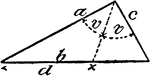### Model Of Geometric Proportions In A Triangle

An illustration showing a model of a triangle that illustrates the following relationship: a:c = d:(b…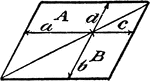### Model Of Geometric Proportions

An illustration showing a quadrilateral model that illustrates the following relationships: a:b = c:d,…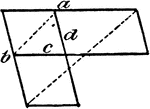### Model Of Geometric Proportions

An illustration showing a model that illustrates the following relationships: a:b = c:d, ad = bc. Product…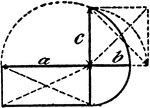### Model Of Geometric Proportions

An illustration showing a model that illustrates the following relationships: a:b = c:b, ab = c²,…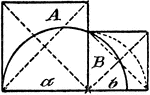### Model Of Geometric Proportions

An illustration showing a model that illustrates the following relationship: A:B = a:b.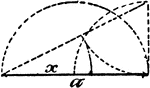### Model Of Geometric Proportions

An illustration showing a model that illustrates the following relationships: a:x = x:a - x, x = √(a²…